All commands, alphabetic order; letter R

\r

The \r command generates a ring accent. The translation of

\r A \r a \r U \r u \r w \r y

is &#xC5; &#xE5; &#x16E; &#x16F; &#x1E98; &#x1E99; or Å å Ů ů ẘ ẙ

The \radical command is valid only in math mode. It is followed by 27-bit number followed by a math field. TeX splits the number into a, f, b and g as it does with any \delimiter. Then it processes the math field and appends a new Rad atom to the current list. The nucleus of this atom contains the specified field; the delimiter field in this atom contains (a, f) and (b, g).

In Tralics, you get an error, a 27-bit number is read and ignored (the number must be between 0 and 134217727 inclusive).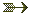(see scanint for how the argument is read)

\raggedbottom

The \raggedbottom command tells LaTeX to use fixed interline space (and variable space at the end of the page), rather than adjust the interline space in order to align the last line of each page (which is the effect of \flushbottom). Both these commands set \@texttop to \relax and \@textbottom to some well chosen value. Unused in Tralics.

\raggedleft, \raggedright

The \raggedleft command starts typesetting in flush-right mode. The \raggedright command starts typesetting in flush-left (non justified) mode.For more information, see description of the command \centering.

\raise

This raises a box.See \moveleft.

\raisebox

Translation of \raisebox{x}[y][z]{\it t} is <raisebox depth='z' height='y' val='x'><hi rend='it'>t</hi></raisebox>. Required argument x, optional arguments y and z are handled in a special way (translation should consist in characters only; it is not possible to fetch the size of the box that contains the last argument). The last argument is evaluated in a group (for instance, font changes are local to the box).

\ra@jobname (Tralics command)

This is like \jobname, but in a case like foo/bar/gee13, the final digits are removed, as well as the directory part. Hence, the result is gee (all characters are of category code 12).

\RAlabel (Tralics command)

The obsolete command \RAlabel reads one argument and does nothing with it.

\rangle (math symbol)

The \rangle command is valid only in math mode. It generates a delimiter (right angle): <mo>&rangle;</mo> (Unicode U+232A, 〉) that renders like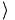.See description of the \vert command.

\RAstartprojet (Tralics command)

This command is not implemented anymore.

RAsection (Tralics environment)

Not a user command. Not implemented anymore. However the commands that start and end a section for the Raweb are still present; they are called \tralics@push@section and \tralics@pop@section.

rawhtml (environment from the html package)

The content of the rawhtml environment is ignored. This environment behaves like the comment environment.For an example, see the description of the latexonly environment.

rawxml (Tralics environment)

When you say \begin{rawxml}, all lines that follow are inserted verbatim in the XML tree as text (be careful with special characters), until a final line that should contain only \end{rawxml}.For an example, see the description of the latexonly environment or the verbatim environment.

\ratio

You can say \ratio{1in}{1cm} if you want a real number as second argument of product and quotient in a calc expression. Both arguments are dimensions.For an example, see \calc.

\ra@year (Tralics command)

The expansion of the command \ra@year is the value of the RA year. This is 2001 if you invoke Tralics with the option year=2001. This is also 2001 if no option is given and current date is between May 2001 and April 2002. This is also 2000 if you translate the activity report of year 2000, case where the file name must be of the form foo2000.tex, and the document class must be ra2000. It is an error if the year option is given and does not match the raweb extension; in non-raweb mode, the year option is ignored if not a number.

\rbrace (math symbol)

The \rbrace command is valid in math mode only; it is then equivalent to a brace \}. Translation is <mo>&rbrace;</mo> (Unicode U+7D).

\rbrack

The \rbrack command is equivalent to (expands to) a bracket ].See also description of the \vert command.

\rceil (math symbol)

The \rceil (right ceiling) command is valid only in math mode. It generates a delimiter: <mo>&rceil;</mo> (Unicode U+2309, ⌉). See also description of the \vert command.

\Re (math symbol)

The \Re command is valid only in math mode. It generates a miscellaneous symbol: <mi>&Re;</mi> (Unicode U+211C, ℜ). See description of the \ldots command.

The \read command is followed by a number, the keyword to, optional spaces and a control sequence (see scanint for details of argument scanning). The command reads a token list and puts it in the control sequence. If the number is valid channel (between 0 and 15), to which a file is associated, the token list will be read from the file; otherwise the token list will be read from the terminal.See \openin for an example.

You can put the prefix \global before \read. In this case the command is globally defined.

The command \readline is an eTeX extension, it has the same syntax as \read, it is followed by a channel number, a to keyword, and a definable command. It reads a line from a file, and puts it in the command. The difference is that all characters are assumed of category code 12, except space that has its standard category code; only one line is read, since the result is always properly nested.

This command takes as argument the name of an XML file, and reads it; the result is put in some special variable. See section manipulating the XML tree for an example.

\real

You can say \real{2.54} or \ratio{1in}{1cm} if you want a real number as second argument of product and quotient in a calc expression. The argument should be such that it produces a dimension when followed by a unit (in the case of \calc{.../\real{1.5}} Tralics reads 1.5pt).For an example, see \calc.

\@reevaluate (Tralics command)

When you say \@reevaluate\foo\bar{gee}, then commands \foo and \bar are called with gee as argument, as in \def\@reevaluate#1#2#3{#1{#3}#2{#3}}. The trick is that you can change category code in the arguments. For details see the titlepage documentation.

\ref

The \ref command takes an argument, which is the name of an anchor (defined by the \label command somewhere in the text), it creates an internal reference to it.See the \label command for example.

The argument is handled in some restricted mode, and the resulting XML converted to a string. As a result, you can say \label{AAAA} and \def\AAA{AAA}\ref{\char65 \AAA}. Contrarily to LaTeX, you get no error about missing \endcsname, even if you say

\ref{\part}

But you get this:

Error signaled at line 5:
End of data reached while scanning argument.
Translating section command div0: <error/>.
Error signaled at line 5:
Error in pop; stack holds div0; trying to pop argument.
Error signaled at line 5:
unexpected element div0.

(the argument of the \part command is missing, the command cannot be the argument of something else, and a division cannot be converted into a string)

\refercite (Tralics command)

This is an extension of the \cite command, explained in in section 2.8 Bibliography of the raweb. The commands \refercite{foo} and \refercite[bar]{foo} are equivalent to \cite[refer][]{foo} and \cite[refer][bar]{foo}.

\refname

This command expands to Bibliography. Its value is used by the thebibliography environment.

\refstepcounter

Expansion of \refstepcounter*{equation} is \stepcounter{equation} plus \tralics@makelabel*{equation}. The optional star is a Tralics feature. See \tralics@makelabel for additional details.

\relax

The translation of \relax is empty. However, in a construct like {\let\foo\relax \xdef\bar{\foo\gee}}, the command \foo is not expanded (since \relax is unexpandable), so that the body of \bar will consists of \foo and the expansion of \gee. In the case of \skip0=2pt\relax plus 5tp, the \relax indicates the end of the glue value (without the \relax, Tralics complains that tp' is not a valid unit). On the other hand, you can say \uppercase \relax{foo}, and the argument to \uppercase is the list {foo}.

\relpenalty (internal integer)

If you say \relpenalty=27, then TeX will use 27 as penalty for line break in a math formula after a relation (like =). This parameter is ignored by Tralics (note that, in the current implementation, $a=b$ and $a\mathbin=b$ are identically translated; it is hence impossible to decide whether the equals sign is a relation or not).(See scanint for details of argument scanning).

\@removeelement

This command takes three arguments A, B and C. Last argument must be a command name or an active character. The second argument is a comma separated list of items, A is removed from B, the result is put in C. If the list B is x, y, z, you must take into account that the second item in the list is not y, but space+y; this means that spaces around commas should be removed first, for instance using zap@space. See an example at \@expandtwoargs

\removelastskip

This command is currently ignored.

\renewcommand

The \renewcommand is like \newcommand. It has the same syntax, but is it used to define commands that are already defined. Here are some examples (note that \relax is not undefined, but you cannot define it).

> \renewcommand{25}{}
Error signaled at line 1:
Attempt to define: 25.
Error signaled at line 1:
Only one token allowed in argument list.
> \renewcommand{$}{} Error signaled at line 2: Cannot define {Character$ of catcode 3}.
> \renewcommand{\Foo}{}
Error signaled at line 3:
\renewcommand: cannot define \Foo; token is undefined.
> \renewcommand{\relax}{}
Error signaled at line 4:
\renewcommand: cannot define \relax; token is undefined.

\renewenvironment

The \renewenvironment is like \newenvironment. It has the same syntax. It is used to modify (redefine) already existing environments. Here are some little examples

> \renewenvironment{xxx}{before}{after}
Error signaled at line 1:
\renewenvironment: cannot define \xxx; token is undefined.
Error signaled at line 1:
\renewenvironment: cannot define \endxxx; token is undefined.
> \let\xx\renewenvironment
> \xx{xxx}{before}{after}
Error signaled at line 3:
\xx: cannot define \xxx; token is undefined.
Error signaled at line 3:
\xx: cannot define \endxxx; token is undefined.
> \newenvironment{xxx}{before}{after}
> \newenvironment{xxx}{before}{after}
Error signaled at line 5:
\newenvironment: cannot define \xxx; token is already defined.
Error signaled at line 5:
\newenvironment: cannot define \endxxx; token is already defined.
> \xx{xxx}{before}{after}

\repeat

The \repeat command is equivalent to \fi, but you should use it to terminate a conditional only in the case of a \loop ...\if ...\repeat construct.See description of \loop.

\RequirePackage

The \RequirePackage command is a synonym of \usepackage. The command takes an optional argument A (options), a required argument B (package name), and an optional argument C (identification). It loads package B (not older than C) with options A.

\RequirePackageWithOptions

The \RequirePackageWithOptions command is like \RequirePackage (see above), it loads package B (not older than C) with options A. The command has only two arguments (B and C), and must be used in a package, from which the option list is inherited.

\restriction (math symbol)

The \restriction command is valid only in math mode. It is an alias for \upharpoonright, translation is <mo>&upharpoonright;</mo> (Unicode U+21BE, ↾).

\resizebox

You can say \resizebox{A}{B}{C}. The result is <resizebox> element containing the translation of the third argument, with attributes height and width that correspond to the translation of the first two arguments. If the argument is an exclamation point, it will be ignored. Otherwise, a dimension is required. Quantities like \width can be used: it refers to the natural width of the box, this is not known to Tralics, and replaced by zero. Example

\resizebox{1in}{2in}{Some text}
\resizebox{1in}{!}{Some text}
\resizebox{1in}{\height}{Some text}

Translation

<resizebox height='144.54pt' width='72.26999pt'>Some text</resizebox>
<resizebox width='72.26999pt'>Some text</resizebox>
<resizebox height='0.0pt' width='72.26999pt'>Some text</resizebox>

\rfloor (math symbol)

The \rfloor (right floor) command is valid only in math mode. It generates a symbol that can be used as a delimiter: <mo>&rfloor;</mo> (Unicode U+230B, ⌋). See also description of the \vert command.

\rfoot

The \rfoot command is valid only if the fancyhdr package is loaded. It takes an optional argument A, and a required argument B (the default value of A is B). The effect is to define the right part of the page footer to A for even pages, to B for odd pages.For more information, see documentation of \fancyhf.

\rgroup (math symbol)

The \rgroup (right group) command is valid only in math mode. It can be used as a delimiter <mo>&#x3015;</mo>, (Unicode U+3015, 〕).

The \rhead command is valid only if the fancyhdr package is loaded. It takes an optional argument A, and a required argument B (the default value of A is B). The effect is to define the right part of the page header to A for even pages, to B for odd pages.For more information, see documentation of \fancyhf.

\rhd (math symbol)

Math symbol, associated to Unicode Character U+22B3, ⊳.

\rho (math symbol)

The \rho command is valid only in math mode. It generates a Greek letter: <mi>&rho;</mi> (Unicode U+3C1, ρ). See description of the \alpha command.

\right

This command is only allowed in a math formula. It has to be followed by a delimiter; moreover you must say \left before \right. For more explanations see \left.

\rightarrow (math symbol)

The \rightarrow command is valid only in math mode. It generates an arrow pointing to the right: <mo>&rightarrow;</mo> (Unicode U+2192, →). See description of the \leftarrow command.

\Rightarrow (math symbol)

The \Rightarrow command is valid only in math mode. It generates an double stroke arrow pointing to the right: <mo>&Rightarrow;</mo> (Unicode U+21D2, ⇒). See description of the \leftarrow command.

\rightarrowtail (math symbol)

The \rightarrowtail command is valid only in math mode. It generates an arrow with tail pointing to the right: <mo>&rightarrowtail;</mo> (Unicode U+21A3, ↣).

\rightarrowtriangle (math symbol)

The \rightarrowtriangle command is valid only in math mode. It generates an arrow: <mo>&roarr;</mo> (Unicode U+21FE, ⇾).

\rightharpoondown (math symbol)

The \rightharpoondown command is valid only in math mode. It generates an arrow pointing to the right, with only the lower part of the arrow-point: <mo>&rightharpoondown;</mo> (Unicode U+21C1, ⇁).

\rightharpoonup (math symbol)

The \rightharpoonup command is valid only in math mode. It generates an arrow pointing to the right, with only the upper part of the arrow-point: <mo>&rightharpoonup;</mo> (Unicode U+21C0, ⇀).

\righthyphenmin (internal integer)

When you say \righthyphenmin=76, then TeX accepts to hyphenate a word only if there are at least 76 characters to the right of the hyphen. Tralics hyphenates nothing.(See scanint for details of argument scanning).

\rightleftarrows (math symbol)

The \rightleftarrows command is valid only in math mode. It generates a pair of arrows pointing to the left and right: <mo>&rightleftarrows;</mo> (Unicode U+21C4, ⇄).

\rightleftharpoons (math symbol)

The \rightleftharpoons command is valid only in math mode. It generates a pair of harpoons pointing to the left and right: <mo>&rightleftharpoons;</mo> (Unicode U+21CC, ⇌).

\rightline

Translation of \rightline{foo} is <line rend='right'>foo</line>See also description of the \centerline command.

\rightmargin (rigid length)

This is the horizontal distance between the right margin of the enclosing environment and the right margin in a list environment. Unused by Tralics.

\rightrightarrows (math symbol)

The \rightrightarrows command is valid only in math mode. It generates a pair of arrows pointing to the right: <mo>&rightrightarrows;</mo> (Unicode U+21C9), ⇉.

\rightskip (rubber length)

You can say \rightskip=10pt plus 2pt minus 3pt. This explains to TeX that it should put 10pt (maybe up to 2pt more, maybe up to 3pt less) of glue on the end (right hand side) of each line. Unused by Tralics (you should use environments like quote or center instead).(See scanglue for details of argument scanning).

\rightslice (math symbol)

The \rightslice command is valid only in math mode. It generates: <mo>&#x2AA7;</mo>, ⪧.

\rightsquigarrow (math symbol)

The \rightsquigarrow command is valid only in math mode. It generates: <mo>&rightsquigarrow;</mo> (Unicode U+21DD, ⇝).

\rightthreetimes (math symbol)

The \rightthreetimes command is valid only in math mode. It generates: <mo>&rightthreetimes;</mo> (Unicode U+22CC, ⋌).

\risingdotseq (math symbol)

The \risingdotseq command is valid only in math mode. It generates: <mo>&risingdotseq;</mo> (Unicode U+2253, ≓).

\rlap

Translation of \rlap{foo} is <line rend='rlap'>foo</line>See also description of the \centerline command.

\rm

The \rm command is equivalent to \normalfont\rmfamily.

In Tralics, a font is defined by a size, a family, a shape, and a series. A command like \rm does not change the size. Available sizes are, in increasing order, tiny, scriptsize, footnotesize, small, normalsize, large, Large, LARGE, huge and Huge. (since v2.13.1, there is also Tiny, SMALL, Small see \@xfontsize). Here is an example

{Aa\tiny Bb %  \smaller Xx \smaller Yy
\scriptsize Cc \footnotesize Dd \small Ee \normalsize Ff
\large Gg \Large Hh \LARGE Ii  \huge Jj  \Huge Kk %\larger Zz
}

The default behavior of Tralics is to use three sizes, small, large and medium, so that the XML result will be:

Aa<hi rend='small'>\>Bb Cc Dd Ee </hi>Ff
<hi rend='large'>Gg Hh Ii Jj Kk</hi>

Preview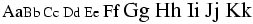But, if the documentclass says useallsizes', or if the configuration file has use_all_sizes = "true", then all ten font sizes are used and the result is the following

Aa<hi rend='small4'>Bb </hi><hi rend='small3'>Cc </hi><hi rend='small2'>Dd </hi>
<hi rend='small1'>Ee </hi>Ff
<hi rend='large1'>Gg </hi><hi rend='large2'>Hh </hi>
<hi rend='large3'>Ii </hi><hi rend='large4'>Jj </hi><hi rend='large5'>Kk</hi>

Preview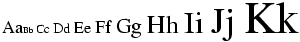If the configuration file contains

use_font_elt = "true"
use_all_sizes = "true"
xml_font_small = "Small"
xml_font_small1 = "Small1"
xml_font_small2 = "Small2"
xml_font_small3 = "Small3"
xml_font_small4 = "Small4"
xml_font_small5 = "Small5"
xml_font_small6 = "Small6"
xml_font_large = "Large"
xml_font_large1 = "Large1"
xml_font_large2 = "Large2"
xml_font_large3 = "Large3"
xml_font_large4 = "Large4"
xml_font_large5 = "Large5"
xml_font_large6 = "Large6"

If we remove the comment before \smaller and \larger and define these adequately (for instance using the amsart document class), we obtain a text with the 13 following sizes

<p>Aa<Small4>Bb </Small4><Small5>Xx </Small5><Small6>Yy </Small6>
<Small3>Cc </Small3><Small2>Dd </Small2><Small1>Ee </Small1>Ff
<Large1>Gg </Large1><Large2>Hh </Large2><Large3>Ii </Large3><Large4>Jj
</Large4><Large5>Kk </Large5><Large6>Zz</Large6></p>

There are some commands that take no argument, reset all font parameters, and change only one of them. Example

{\rm Some text} {\sf Some text} {\tt Some text}
{\bf Some text} {\it Some text} {\sc Some text} {\sl Some text}

This is the resulting XML

Some text <hi rend='sansserif'>Some text</hi> <hi rend='tt'>Some text</hi>
<hi rend='bold'>Some text</hi> <hi rend='it'>Some text</hi> <hi rend='sc'>Some text</hi> <hi rend='it'>Some text</hi>

Preview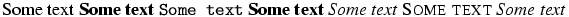There are commands that change only one parameter. For instance \rmfamily and \textrm. The first command is a declaration, the second takes an argument. The family can be one of rm, sf or tt (roman, sans-serif and typewriter). The series can be md or bf (medium or bold face), and the shape can be up, it, sl, or sc (upright, italic, slanted or small-caps). This makes 24 possibilities (the product). In the example below, we show these 24 lines. On each cases there are 11 commands (3 family selections, 2 series selection, 4 shape selections, plus the \textnormal and \emph). This gives a total of 264 cases.

\def\Test{
\textrm{Textrm} \textsf{Textsf} \texttt{Texttt}
\textbf{Testbf} \textit{Textit} \textsc{Textsc} \textsl{Textsl}
\textnormal{Textnormal} \textmd{Textmd} \emph{Emph} \textup{Textup}
\par}

{\rmfamily \mdseries \upshape rmmdup \Test}
{\sffamily \mdseries \upshape sfmdup \Test}
{\ttfamily \mdseries \upshape ttmdup \Test}
{\rmfamily \bfseries \upshape rmbfup \Test}
{\sffamily \bfseries \upshape sfbfup \Test}
{\ttfamily \bfseries \upshape ttbfup \Test}

{\rmfamily \mdseries \itshape rmmdit \Test}
{\sffamily \mdseries \itshape sfmdit \Test}
{\ttfamily \mdseries \itshape ttmdit \Test}
{\rmfamily \bfseries \itshape rmbfit \Test}
{\sffamily \bfseries \itshape sfbfit \Test}
{\ttfamily \bfseries \itshape ttbfit \Test}

{\rmfamily \mdseries \scshape rmmdsc \Test}
{\sffamily \mdseries \scshape sfmdsc \Test}
{\ttfamily \mdseries \scshape ttmdsc \Test}
{\rmfamily \bfseries \scshape rmbfsc \Test}
{\sffamily \bfseries \scshape sfbfsc \Test}
{\ttfamily \bfseries \scshape ttbfsc \Test}

{\rmfamily \mdseries \slshape rmmdsl \Test}
{\sffamily \mdseries \slshape sfmdsl \Test}
{\ttfamily \mdseries \slshape ttmdsl \Test}
{\rmfamily \bfseries \slshape rmbfsl \Test}
{\sffamily \bfseries \slshape sfbfsl \Test}
{\ttfamily \bfseries \slshape ttbfsl \Test}

The result is a bit longish. You can see it here. Preview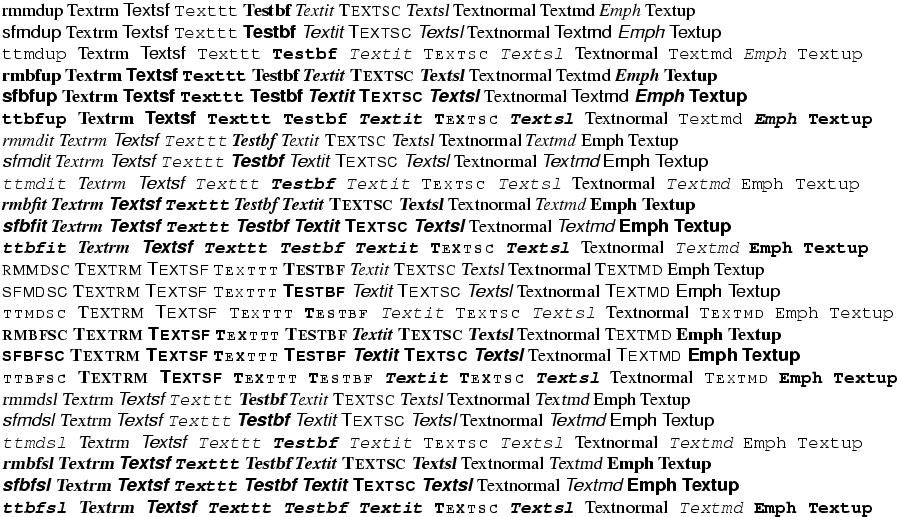The next example shows that you can use some math constructs in text mode.

1\textsuperscript{st} x\textsuperscript{ième} y\textsubscript{some text}.
$1^e$ \overline{foo}\underline{bar}

The translation is

1<sup>st</sup> x<sup>ième</sup> y<sub>some text</sub>
1<sup>e</sup><overline>foo</overline><underline>bar</underline>.

Preview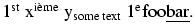\rmfamily

The \rmfamily command changes the family of the current font to roman family.For an example of fonts, see \rm.

\rmoustache (math symbol)

The \rmoustache command is valid only in math mode. It generates a closing delimiter: <mo>&rmoustache;</mo> (Unicode U+23B1, ⎱).

\roman, \@roman

The \roman command takes as argument a counter (see counters in latex), and typesets its value using lower case roman characters.For an example see the \alph command.

The expansion of \roman{foo} is \romannumeral\c@foo. The expansion is empty if the number is zero or negative.
\@roman is the same as \romannumeral.

\Roman, \@Roman

Like \roman above, result is uppercase. \@Roman is the same as \Romannumeral.

\romannumeral

The \romannumeral command converts a number using Roman lower case digits. It calls scanint in order to get a number. The expansion is a sequence of letters (of catcode 12). The expansion is empty if the number is zero or negative. The expansion of \romannumeral 1984 is mcmlxxxiv. The following piece of code verifies this. We use the \catletter command in order to change catcodes, and another auxiliary command (replacing \somecats by its body does not work).

\def\catletter#1{\catcode#1=12 }
\def\somecats{\catletter\m\catletter\c\catletter\x\catletter\i
\catletter\v\catletter\l}
{\somecats \gdef\Testmcmlxxxiv{}}
\expandafter\Test\romannumeral 1984

See the \csname command for a funny use of \romannumeral. The idea being that it expand N thousands to a list of N character tokens. It is hence possible to repeat something N times (with pure expansion).

\Romannumeral

Like \romannumeral above, result is uppercase. Similar example.

\def\catletter#1{\catcode#1=12 }
\def\somecats{\catletter\M\catletter\C\catletter\X\catletter\I
\catletter\V\catletter\L}
{\somecats \gdef\TestMCMLXXXIV{}}
\expandafter\Test\Romannumeral 1984

Note that LaTeX implements \Roman via \romannumeral and a recursive function that converts lowercase to uppercase. In the following piece of code, \Testa holds mcmlxxxiv' (because the page counter is \count0) and \Testb shows x, because these characters are of \catcode 12. In LaTeX, \TestA holds MCMLXXXIV' (catcode letter) and \TestB shows C (whereas Tralics shows X).

\count0=1984
\edef\Testa{\roman{page}}
\edef\TestA{\Roman{page}}
\def \Testb #1m#2{\show #2}
\def \TestB #1M#2{\show #2}
\expandafter\Testb\Testa mx
\expandafter\TestB\TestA MX

\root

The \sqrt command puts a square root sign over its argument. It can take an optional argument, and \sqrt[x]{y} is equivalent to \root x \of{y}. It works only in math mode.See also the \acute command.

\rotatebox

The \rotatebox command takes two arguments, the first is an attribute value (it should be an angle), and the second is a box content (a priori paragraphs are not allowed). For an example, see \fbox. If the graphicx package is loaded, you can say \rotatebox[x=1pt,y=2pt,origin=c,units= -360] {30} {Text}. In this case the translation is <pic-rotatebox units='-360' origin='c' y='2.0pt' x='1.0pt' angle='30'>Text</pic-rotatebox>

\rowattribute (Tralics commmand)

If you say \rowattribute{mathvariant}{bold}, this adds the attribute mathvariant='bold' to the current row. The command can be used only in math mode; you should not use this command outside a table (or a construction like the align environment that produces a table). For an example of use, see \mathattribute.

\rq

The \rq command expands to a right quote (single-quote). You can say \lq\foo\rq' or `\foo'', the result is the same.

\rrbracket (constant)

The \rrbracket command is valid only in math mode. It generates double stroke bracket <mo>&RightDoubleBracket;</mo> (Unicode U+301B, 〛).

\rrparenthesis (constant)

The \rrparenthesis command is valid only in math mode. It generates a duble stroke parenthesis: <mo>&#x2988;</mo>, ⦈.

\Rrightarrow (constant)

The \Rrightarrow command is valid only in math mode. It generates a triple stroke arrow pointing to the right: <mo>&Rrightarrow;</mo> (Unicode U+21DB, ⇛).

\rrrt (Tralics command)

The command \rrrt{foo} is a short-hand for \href{http://www.inria.fr/rrrt/foo.html}.

\Rsh (math symbol)

The \Rsh command is valid in math mode only; it produces the symbol <mo>&Rsh;</mo> (Unicode U+21B1, ↱).

\rtimes (math symbol)

The \Rsh command is valid in math mode only; it produces the symbol <mo>&rtimes;</mo> (Unicode U+22CA, ⋊).

\rule

The command \rule[A]{B}{C} takes three arguments, the default value of the first argument is zero. It leaves vertical mode and produces a rule of width B and height C; the rule is shifted by A. Example, assuming the calc package loaded.

\rule{2pt}{3pt}
\rule[1pt]{3pt+5pt}{17PT}

Translation

<rule width='2.0pt' depth='0.0pt' height='3.0pt'/>
<rule width='8.0pt' depth='-1.0pt' height='18.0pt'/>

\rvert (math symbol)

The \rvert command is valid in math mode only; it is a right delimiter associated to \vert. it produces the symbol <mo>&vert;</mo> (Unicode U+7C, |).

\rVert (math symbol)

The \rVert command is valid in math mode only; it is a right delimiter associated to \Vert. it produces the symbol <mo>&parallel;</mo> (Unicode U+2225, ∥).back to home page © INRIA 2003-2005, 2006 Last modified $Date: 2015/12/01 17:24:43$